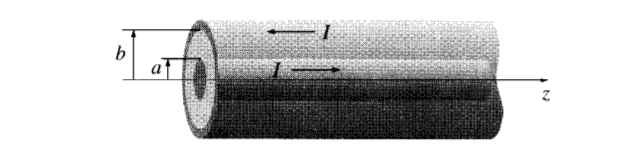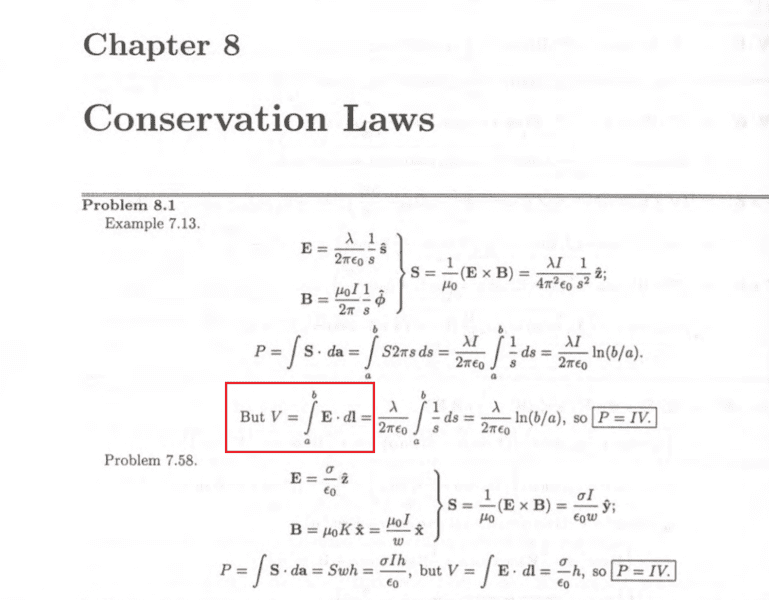# Problem about electric potential and power transmitted down a cable

• Another

#### AnotherWhen to use ## V =-\int E ⋅ dl ## or ## V =\int E ⋅ dl ## ?

When i use ## V =-\int E ⋅ dl ##. i can not show that P = IV because ##\frac{ln \frac{b}{a}}{ln \frac{a}{b}} ≠ 1##

But the solution use ## V =\int E ⋅ dl ## I'm concerned about using "minus" .

#### Attachments

The notation in the solutions is kind of sloppy, but it's easy enough to understand what was meant.

Assume that ##\vec E## points radially outward. Then the inner conductor is at a higher potential than the outer conductor so that ##V = V_a - V_b##. So you have
$$V = V_a - V_b = -\int_b^a \vec E \cdot d\vec l = \int_a^b \vec E \cdot d \vec l.$$

•Another